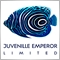Meta trader crashed on calculation27

hi guys,

may you help me on this code please :

string total_modes()

{

string ret="";

int sum=0;

ulong i=0;

int interest=1;

bool allnotfull=false;

for(int y=1;y<=currencynumber;y++) currencyinerest[y]=interest;

while(sum<currencynumber*100)

{

currencyinerest[currencynumber]+=1;

sum=0;

for(int y=1;y<=currencynumber;y++)

{

sum=sum+currencyinerest[y];

}

if(sum==100)

{

i++;

}

for(int y=currencynumber;y>=2;y--)

if(currencyinerest[y]==100)

{

currencyinerest[y]=0;

currencyinerest[y-1]=currencyinerest[y-1]+1;

}

}

ret="Calculation On "+IntegerToString(currencynumber)+" Currency with " +IntegerToString(i)+" Modes.";

return ret;

}

this function return the number of modes that sum of all variables(currencyinerest+currencyinerest+...+curencyinerest[n]) are equal to 100;

this code perfectly work when you assign number 3 to to variable "currencynumber"

when you assign number 4 to "currencynumber" the code works with some delay, but if you assign a number greater than 4 to "currencynumber" meta trader will crashed.

i really need to run it on 20 currency , and i know it will takes several minutes to calculate;so what's your offer?

any suggestion will much appreciated.Moderator
9085

Please use the </> button to insert your above code.38981

Farnood1988:

hi guys,

this function return the number of modes that sum of all variables(currencyinerest+currencyinerest+...+curencyinerest[n]) are equal to 100;

this code perfectly work when you assign number 3 to to variable "currencynumber"

when you assign number 4 to "currencynumber" the code works with some delay, but if you assign a number greater than 4 to "currencynumber" meta trader will crashed.

i really need to run it on 20 currency , and i know it will takes several minutes to calculate;so what's your offer?

any suggestion will much appreciated.

Of course it "crashed". For currencynumber=3 the while loop is executed ~100^3.

So for 5 it's 100^5 = 10,000,000,000, for 20 it will be 10,000,000,000,000,000,000,000,000,000,000,000,000,000...that's insane :-D

Your "bruteforce" algorithm is not usable. You need to find an other one. And the worst is it's not even correct for 3 (you need currencyinerest[x] to be >0, right ?).27

Alain Verleyen:

Of course it "crashed". For currencynumber=3 the while loop is executed ~100^3.

So for 5 it's 100^5 = 10,000,000,000, for 20 it will be 10,000,000,000,000,000,000,000,000,000,000,000,000,000...that's insane :-D

Your "bruteforce" algorithm is not usable. You need to find an other one. And the worst is it's not even correct for 3 (you need currencyinerest[x] to be >0, right ?).

dear Alain

thanks for reply.As you said, i know exactly, how similar the code of function is to the code of insanity. :-)))))

but i really need to calculate it , and i'm pretty sure there is solution for it; because solver software's can solve this problem in few moments.so do you have any idea how to do it in Mql?27

Eleni Anna Branou:

Please use the </> button to insert your above code.

Dear Eleni

it was really useful.thank you.:)38981

Farnood1988:

dear Alain

thanks for reply.As you said, i know exactly, how similar the code of function is to the code of insanity. :-)))))

but i really need to calculate it , and i'm pretty sure there is solution for it; because solver software's can solve this problem in few moments.so do you have any idea how to do it in Mql?